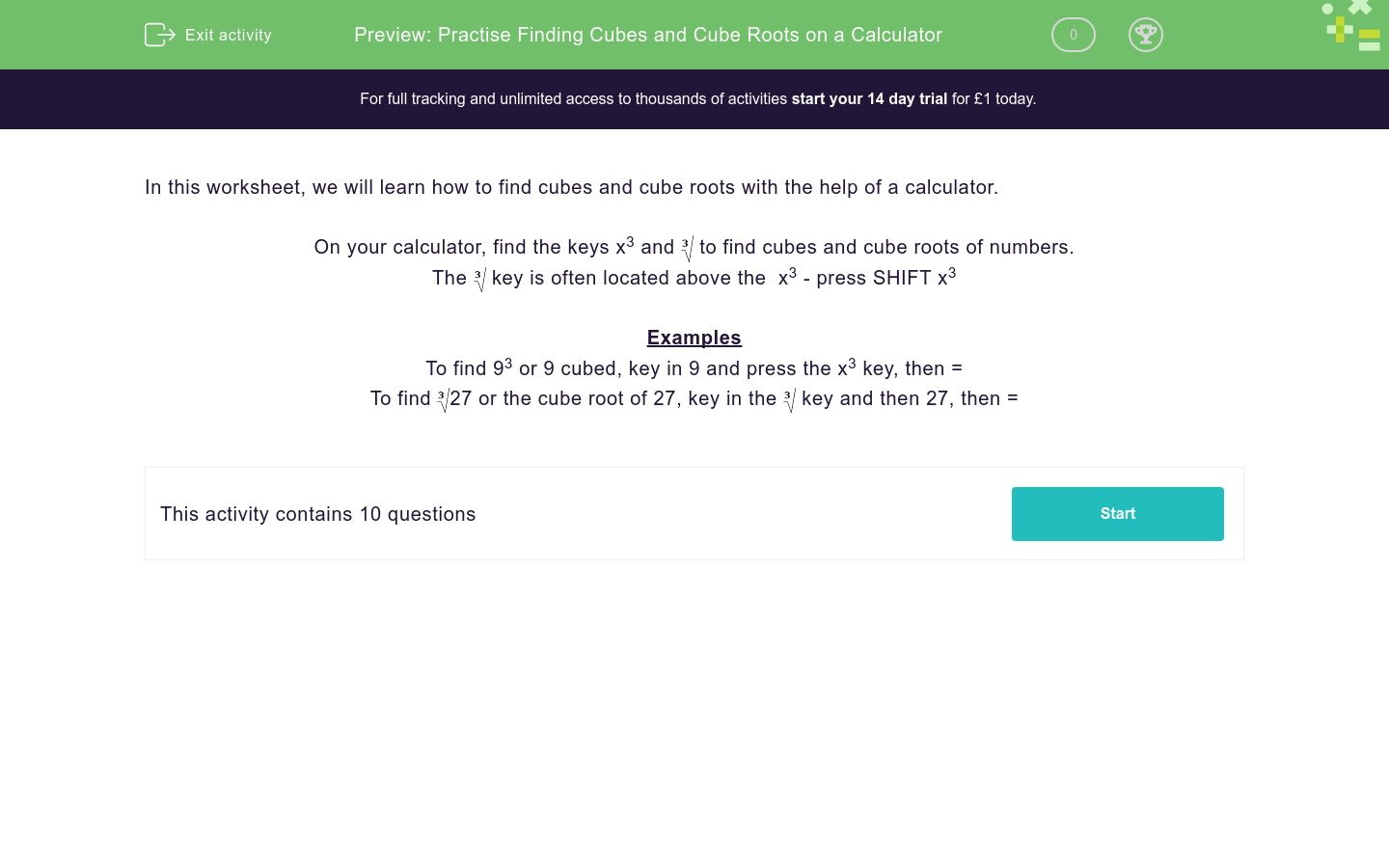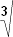# Practise Finding Cubes and Cube Roots on a Calculator

In this worksheet, students use a calculator to find cubes and cube roots.Key stage:  KS 3

Curriculum topic:   Number

Curriculum subtopic:   Use Calculators/Technology for Accuracy

Difficulty level:### QUESTION 1 of 10

In this worksheet, we will learn how to find cubes and cube roots with the help of a calculator.

On your calculator, find the keys x3 andto find cubes and cube roots of numbers.

Thekey is often located above the  x3 - press SHIFT x3

Examples

To find 93 or 9 cubed, key in 9 and press the x3 key, then =

To find27 or the cube root of 27, key in thekey and then 27, then =

Find the value of:

633 = _________

Find the value of:

183 = _________

Find the value of:729  =
 _________

Find the value of:583  =
 _________

Find the value of:18  =
 _________

Find the value of:981  =
 _________

Find the value of:576  =
 _________

Find the value of:

8.53

Find the value of:626  =
 _________

Find the value of A.A  =
 8
• Question 1

Find the value of:

633 = _________

250047
• Question 2

Find the value of:

183 = _________

5832
• Question 3

Find the value of:729  =
 _________
9
• Question 4

Find the value of:583  =
 _________

8.4
• Question 5

Find the value of:18  =
 _________

2.6
• Question 6

Find the value of:981  =
 _________

9.9
• Question 7

Find the value of:576  =
 _________

8.3
• Question 8

Find the value of:

8.53

614.125
• Question 9

Find the value of:626  =
 _________

8.6
• Question 10

Find the value of A.A  =
 8
512
EDDIE SAYS
Find the cube of 8
---- OR ----

Sign up for a £1 trial so you can track and measure your child's progress on this activity.

### What is EdPlace?

We're your National Curriculum aligned online education content provider helping each child succeed in English, maths and science from year 1 to GCSE. With an EdPlace account you’ll be able to track and measure progress, helping each child achieve their best. We build confidence and attainment by personalising each child’s learning at a level that suits them.

Get started